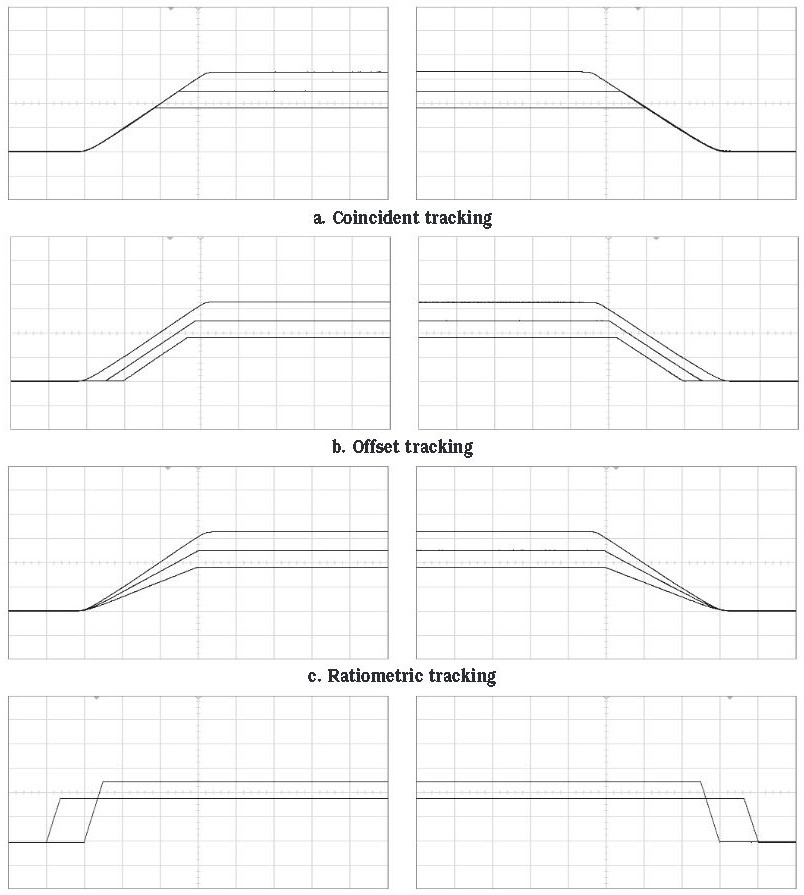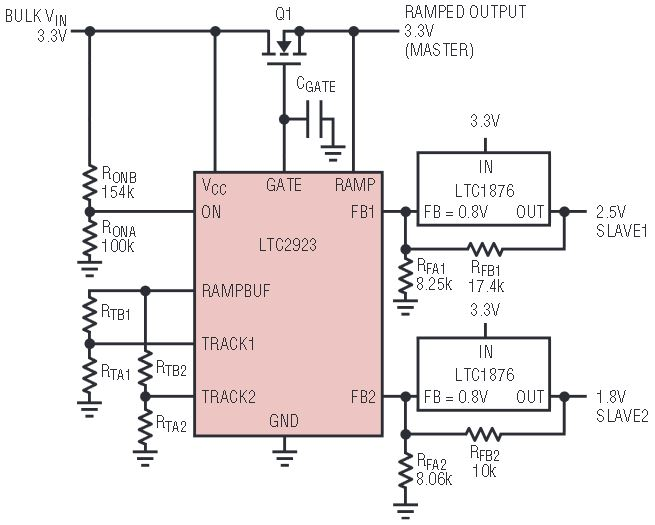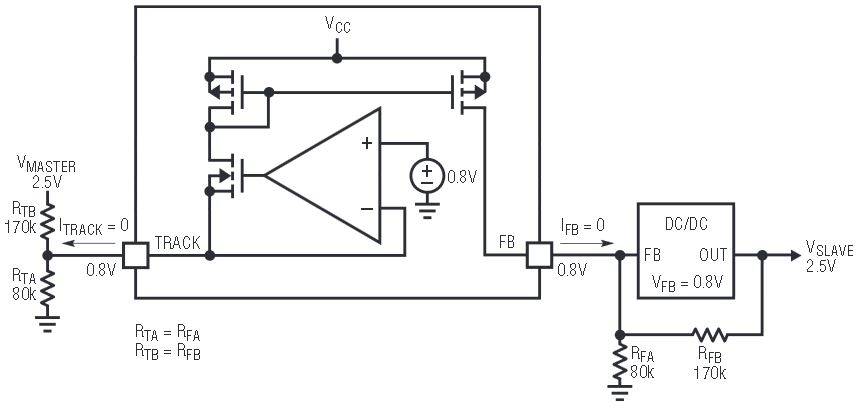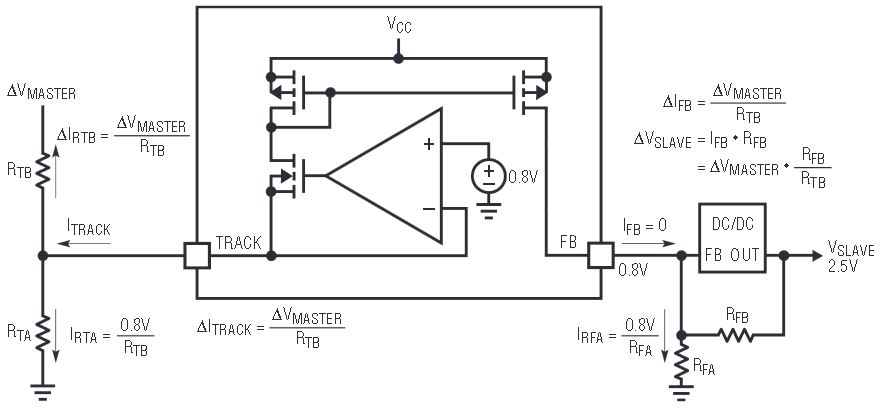# Versatile Power Supply Tracking without MOSFETs

Introduction

Modern electronics systems often have complex power supply voltage tracking and sequencing requirements. Ignoring these requirements can result in the immediate destruction of devices, or worse yet, premature failures in the field.

Voltage tracking requirements usually specify that the voltage difference between two power supplies must never exceed a certain limit. This constraint applies at all times: during power up, power down, and steady state operation.

In contrast, supply sequencing requirements specify the order in which supplies power up and power down.

Figure 1 shows various tracking and sequencing scenarios.Figure 1. Types of power supply voltage tracking.

The penalty for poor tracking or sequencing is often irreparable damage to devices in the system. FGPAs, PLDs, DSPs and microprocessors typically have diodes placed between the core and I/O supplies as a component of the ESD protection. If the supplies violate the tracking requirements and forward bias the protection diodes, the device may be damaged.

Forward biasing internal diodes, whether protection diodes or other diodes inherent to the CMOS process, may trigger latchup destroying the device. In other cases, when the I/O supply rises before the core supply, undefined logic states in the core can cause excessive current in the I/O circuitry. Even when the individual components of a system do not require supply tracking or sequencing, the complete system may require power supply sequencing for proper operation. For example, a system clock may need to start before a block of logic is powered.

A Simple and Versatile Solution

The LTC2923 provides a simple and versatile solution to both power supply tracking and sequencing without the drawbacks of series MOSFETs.

By selecting a few resistors, the supplies are configured to ramp-up and ramp-down with a variety of voltage profiles. Tracking requirements can dictate that supply voltages are equal during ramp up and ramp down (Figure 1a), or that the supplies must ramp up and down with fixed voltage offsets between them (Figure 1b), or that they must ramp up and down ratiometrically (Figure 1c). Alternatively, supply sequencing may be required, which can also be handled by the LTC2923 (Figure 1d).

Many voltage tracking solutions use series MOSFETs, which have the problems of an inherent voltage drop, additional power consumption, and extra PC board real estate.

Instead, the LTC2923 controls supplies by injecting current into their feedback nodes and thus avoids the pass element losses inherent in series MOSFET solutions. Furthermore, power supply stability and transient response remain unaffected because the injected current from the LTC2923 offsets the output voltage without altering the power supply control loop dynamics.

Power supply tracking is straightforward with the LTC2923. It controls two slave supplies that track a master signal. A pair of resistors configures the behavior of each slave supply relative to the master signal. The choice of resistors can cause a slave supply to track the master signal exactly or with a different ramp rate, voltage offset, time delay, or combination of these.

An optional series FET provides support for a third supply or any supply that does not allow direct access to its feedback resistors, such as module power supplies, as shown in Figure 2. When the optional series FET is used, its output serves as the master signal. If the series FET is not used, the master signal is generated by tying a capacitor from the GATE and RAMP pins to ground as shown in Figure 3.Figure 2. Typical coincident tracking application. The optional series FET is used to ramp the 3.3V bulk supply.Figure 3. If the series FET is not used, the master signal is generated by tying a capacitor from the GATE and RAMP pins to ground.

Tracking and/or Sequencing Power Supplies is as Easy as 1, 2, 3

Any of the profiles shown in Figure 1 can be achieved by using the following simple design procedure. Figure 2 shows a basic three-supply application circuit.

1. Set the ramp rate of the master signal.
Solve for the value of CGATE, the capacitor on the GATE pin, based on the desired ramp rate (V/s) of the master supply, SM.
If the external FET has a gate capacitance comparable to CGATE, then the external capacitor’s value should be reduced to compensate for the FET’s gate capacitance.
If no external FET is used, tie the GATE and RAMP pins together.
2. Solve for the pair of resistors that provide the desired ramp rate of the slave supply, assuming no delay.
Choose a ramp rate for the slave supply, SS. If the slave supply ramps up coincident with the master supply or with a fixed voltage offset, then the ramp rate equals the master supply’s ramp rate. Be sure to use a fast enough ramp rate for the slave supply so that it will finish ramping before the master supply has reached its final supply value. If not, the slave supply will be held below the intended regulation value by the master supply. Use the following formulas to determine the resistor values for the desired ramp rate, where RFB and RFA are the feedback resistors in the slave supply and VFB is the feedback reference voltage of the slave supply:
Note that large ratios of slave ramp rate to master ramp rate, SS/SM, may result in negative values for RTAʹ. If a sufficiently large delay is used in step 3, RTA will be positive, otherwise SS/SM must be reduced.
3. Choose RTA to obtain the desired delay.
If no delay is required, such as in coincident and ratiometric tracking, then simply set RTA = RTAʹ. If a delay is desired, as in offset tracking and supply sequencing, calculate RTAʹʹ to determine the value of RTA where tD is the desired delay in seconds.
the parallel combination of RTAʹ and RTAʹʹ
As noted in step 2, small delays and large ratios of slave ramp rate to master ramp rate (usually only seen in sequencing) may result in solutions with negative values for RTA. In such cases, either the delay must be increased or the ratio of slave ramp rate to master ramp rate must be reduced.

How a Simple Cell Tracks and Sequences Supplies

The operation of the LTC2923 is based on the simple tracking cell shown in Figure 4. This cell servos the TRACK pin to 0.8V and mirrors the current supplied by that pin at the FB pin. Connecting a resistive divider from the master signal to the TRACK pin configures the FB pin’s current as a function of the master signal. By selecting the appropriate resistor values, RTA and RTB, it is possible to generate any of the profiles shown in Figure 1.Figure 4. Simplified tracking cell.

The LTC2923’s data sheet outlines a simple 3-step procedure to choose resistor values for each of the supply behaviors shown in Figure 1. This procedure is described in the data sheet and reprinted in the sidebar here. The rest of this section covers a more in-depth analysis of the operation of the LTC2923.

Examine the schematic shown in Figure 5, and assume that the DC/DC converter’s feedback voltage is 0.8V.Figure 5. IFB forces VSLAVE to 0V.

Now, consider the case where VMASTER = 0V. Here, the current from the TRACK pin flows through RTA and RTB, which are both grounded. The tracking cell mirrors this same current at the FB pin. Because RTA = RFA and RTB = RFB, 0.8V is forced at the feedback node of the DC/DC converter. This results in an output voltage, VSLAVE, of 0V. Any voltage greater than 0V at the DC/DC converter’s output results in a feedback voltage greater than 0.8V causing the converter to drive its output towards 0V. So, with RTA = RFA and RTB = RFB, VSLAVE = 0V when VMASTER = 0V.

Now, consider the case in Figure 6 where VMASTER = 2.5V, the nominal output voltage of the DC/DC converter. Without the LTC2923, the DC/DC converter’s feedback network drives 0.8V at its feedback node while generating an output voltage of 2.5V. Since RTA = RFA and RTB = RFB, the resistive divider formed by RTA and RTB forces 0.8V at the TRACK pin when VMASTER = 2.5V, without requiring any current from the tracking cell. Since the tracking cell only sources current, no current flows from the TRACK pin when VMASTER ≥ 2.5V. Therefore, when VMASTER is above 2.5V the DC/DC converter operates as if the tracking cell were not present.Figure 6. VSLAVE = 2.5V when VMASTER = 2.5V.

The relationship between VMASTER and VSLAVE is linear between 0V and 2.5V, so the fact that the DC/DC converter’s output is equal to VMASTER at 0V and at 2.5V means that VMASTER and VSLAVE are equal at all points in between. The DC/DC converter’s output tracks VMASTER exactly until VMASTER rises above 2.5V. When VMASTER is above 2.5V, no current flows from the FB pin and the tracking cell is effectively removed from the circuit.

The fact that RTA = RFA and RTB = RFB in this example is not just a fortunate coincidence. For a DC/DC converter with a feedback voltage of 0.8V, setting the track resistors, RTA and RTB, equal to the feedback resistors, RFA and RFB causes the supplies to track together exactly. When the feedback voltage is not equal to 0.8V, only RTA needs to be adjusted, a result that is discussed below.

RTB Configures the Ramp Rate

The LTC2923 really shows flexibility when RTA ≠ RFA and/or RTB ≠ RFB. First consider the effects of RTB’s value.

RTB configures the ramp rate of the slave supply. More accurately, RTB configures the gain of the slave supply relative to the master supply and ultimately determines the ramp rate. Continuing with the example in Figure 5, as long as the track cell is regulating the TRACK pin at 0.8V (which is true for VMASTER < 2.5V), the current through RTA is fixed at 0.8/RTA. Therefore, the current change in response to a voltage change at VMASTER is completely determined by RTB, as shown in Figure 7. Because one end of the resistor RTB is fixed at 0.8V, the change in current from the TRACK pin is equal toFigure 7. RTB configures gain (ramp rate).

This change in current is mirrored at the FB pin. Since the DC/DC converter regulates its feedback node to 0.8V, the voltage across RFA is fixed at 0.8V, and thus the current through RFA is also fixed. Any change in the current from the FB pin results in an equal change in the current across RFB. If RTB = RFB then any change in voltage at VMASTER results in an equal change in the voltage at the DC/DC converter’s output, VSLAVE. If RFB is increased relative to RTB the voltage change at VSLAVE is greater than the voltage change at VMASTER. The change in voltage at the DC/DC converter’s output is:

This can be interpreted as a gain of RFB/RTB between the master signal and the output voltage.

Because an external capacitor connected to the GATE pin programs the master supply’s ramp rate at:

the ramp rate of the slave supply is:

RTA Corrects for Converter VREF

As mentioned above, RTA is adjusted when a DC/DC converter’s feedback voltage is not equal to 0.8V. If, for example, the DC/DC converter’s feedback voltage is 1.2V, then the current from the FB pin, IFB, needed to hold the converter’s output at 0V is

RTB = RFB still sets the gain between VMASTER and VTRACK to 1V/V. If VMASTER is at 0V, then ITRACK just barely holds VSLAVE at 0V when

Solving for RTA gives the exact value of RTA that compensates for the 1.2V feedback voltage as opposed to the 0.8V value.

This value of RTA, the value that sets VSLAVE to track VMASTER with no delay, is represented by RTAʹ in the 3-step design procedure.

RTA Configures an Offset

RTA not only compensates for the DC/DC converter’s feedback voltage, it serves an even broader purpose. It configures an offset voltage between VMASTER and VSLAVE.

To demonstrate this, assume that RTA is reduced to 62kΩ so that RTA < RFA as shown in Figure 8. This creates the voltage profile shown in Figure 9. Compared to the previous example, more current flows from the TRACK pin when VMASTER is at ground. The output of the DC/DC converter remains at ground until VMASTER rises high enough to reduce the current from the TRACK pin to the level that just holds VSLAVE at 0V:Figure 8. RTA < RFA configures offset tracking.Figure 9. Output profile of circuit in Figure 8.

The gain between VSLAVE and VMASTER is still determined by RFB/RTB as VSLAVE ramps from 0V to 2.5V, and the voltage across RTA is still 0.8V, so the ramp rate does not vary with RTA. VSLAVE, however, does not rise above 0V until VMASTER reaches 0.5V in this example.

In the 3-step design procedure, the slave supply is offset by RTA˝, the value of resistor that is placed in parallel with RTAʹ to produce the desired offset.

RTA Configures a Delay

Alternatively, the voltage offset generated by RTA may be interpreted as a time delay between the supplies. In the above example it was shown that VSLAVE remains at ground until VMASTER rises above 0.5V. Since the ramp-rate is 1000V/s and VSLAVE follows 0.5V below VMASTER, this can also be interpreted as a time delay of

This representation is helpful when implementing supply sequencing. To sequence supplies, the slave supplies are ramped up at a faster rate than the master signal and each is delayed.

By choosing the appropriate ramp rates and time delays, each supply is completely powered before the next supply ramps up. Multiple iterations may be necessary to find an acceptable solution since small delays combined with large slave ramp rate to master ramp rate ratios may result in negative values for RTA. Also, the chosen values for RTA and RTB must not require more than 1mA from the TRACK pin.

In the 3-step design procedure this delay is achieved with RTA˝, the value of resistor that is placed in parallel with RTAʹ to produce the desired delay.

Examples

Now consider how varying RTA and RTB can produce each of the voltage tracking profiles in Figure 1. The schematic in Figure 2 uses an LTC1876 dual synchronous step-down converter to produce 2.5V and 1.8V supplies from a 3.3V input. An LTC2923 connected to the feedback nodes controls the ramp-up and ramp-down behavior of these supplies. In the following example, the 3-step design procedure outlined in the sidebar is followed.

An external Si2306 MOSFET controls the behavior of the 3.3V supply with the ramp-rate determined by the capacitor on the GATE pin. Following Step 1 of the 3-Step design procedure, if a ramp-rate, SM, of 1000V/s is desired, equation (1) results in:

If ramp-rate accuracy is critical then the external MOSFET’s gate capacitance should be subtracted from the CGATE capacitor’s value. If no MOSFET is used, tie the GATE and RAMP pins together and connect the CGATE capacitor between those pins and ground.

Coincident Tracking

Consider coincident tracking as shown in Figure 1a. We know from the above discussion that if the feedback voltage of the switching power supply is 0.8V, as it is on the LTC1876, then coincident tracking can be configured by setting the tracking resistors equal to the feedback resistors. In this case,

Verify this conclusion by following Step 2 of the 3-Step Design Procedure. From equation (2):

And from equation (3):

As mentioned above, in the 3-step design procedure RTAʹ represents the value of RTA that produces no delay or offset. Since no delay is desired, RTA1 = RTA1ʹ and RTA2 = RTA2ʹ, and Step 3 of the Design Procedure is unnecessary.

Ratiometric Tracking

Now consider ratiometric tracking as seen in Figure 1b. Set the slave ramp-rates, SS1 and SS2:

Round up the ramp-rates, SS1 and SS2, to 800V/s and 600V/s so that there is no chance of the 3.3V supply voltage holding the 2.5V or 1.8V supplies low after the ramp-up is complete.

Solving equation (3) from Step 2 of the Design Procedure:

This agrees with the intuitive expectation that RTB1 and RTB2 should be less than RFB1 and RFB2, because we desire gains of less than 1V/V between the master and the slave supplies. (Remember that the gain is RFB/RTB.)

To complete Step 2, we must also solve for RTA1ʹ and RTA2ʹ using equation (3).

Since no delay is desired, RTA1 = RTA1ʹ and RTA2 = RTA2ʹ and Step 3 is unnecessary.

Offset Tracking

In this example, the 2.5V supply ramps up 0.5V below the 3.3V supply, and the 1.8V supply ramps up 1V below the 3.3V supply, as shown in Figure 1c. In offset tracking, the ramp-rates are equal so the gain between the master and slave supplies is 1V/V. As in Step 1 of the coincident tracking example:

Remember that RTA is used to configure the offsets. We know intuitively that RTA1 and RTA2 should be smaller than in the coincident tracking example to generate offsets in the slave outputs. We already found that

in the coincident tracking example above. Now we can use Step 3 of the Design Procedure to solve for RTA1 and RTA2 after converting the voltage offset to a time delay.

From equation (4):

Remember that RTA˝ is the value of resistor that is placed in parallel with RTAʹ to produce the desired offset. Therefore, RTA1 and RTA2 can be found from equation (5):

Supply Sequencing

To produce a voltage profile such as the one shown in Figure 1d, supply sequencing applications require the slaves to have fast ramp rates and time delays relative to the master. First, reducing the master signal ramp-rate to 100V/s using the equation from Step 1 of the Design Procedure results in:

Set the slave ramp rates to 500V/s in equation (2) to find RTB1 and RTB2:

Intuitively, RFB/RTB should be 5 times greater than the previous examples since the gain from the master signal to the slave supply is now 5 times greater.

Complete Step 2 by solving for RTA1ʹ and RTA2ʹ using equation (3).

Step 3 adjusts RTA1 and RTA2 for the desired delays between the two supplies. In this case, factor in a delay of 20ms for the 2.5V supply relative to the master signal and a delay of 10ms for the 1.8V supply.

Note that not every combination of ramp-rates and delays is possible. Small delays and large ratios of slave ramp rate to master ramp rate may result in solutions that require negative resistors. In such cases, either the delay must be increased or the ratio of slave ramp rate to the master ramp rate must be reduced. In addition, the chosen resistor values should not require more than 1mA to flow from the TRACK and FB pins. Therefore, confirm that less than 1mA flows from TRACK when VMASTER is at 0V.

Conclusion

The LTC2923 simplifies power supply tracking and sequencing while offering superior performance in a smaller area than competing solutions. A few resistors can configure simple or complex supply behaviors. In most cases, series MOSFETs are eliminated along with their parasitic voltage drops and power consumption, but for those designs that require a series MOSFET, support is provided for one. The LTC2923 offers all of these features in a tiny MS10 or leadless 12-pin DFN package.